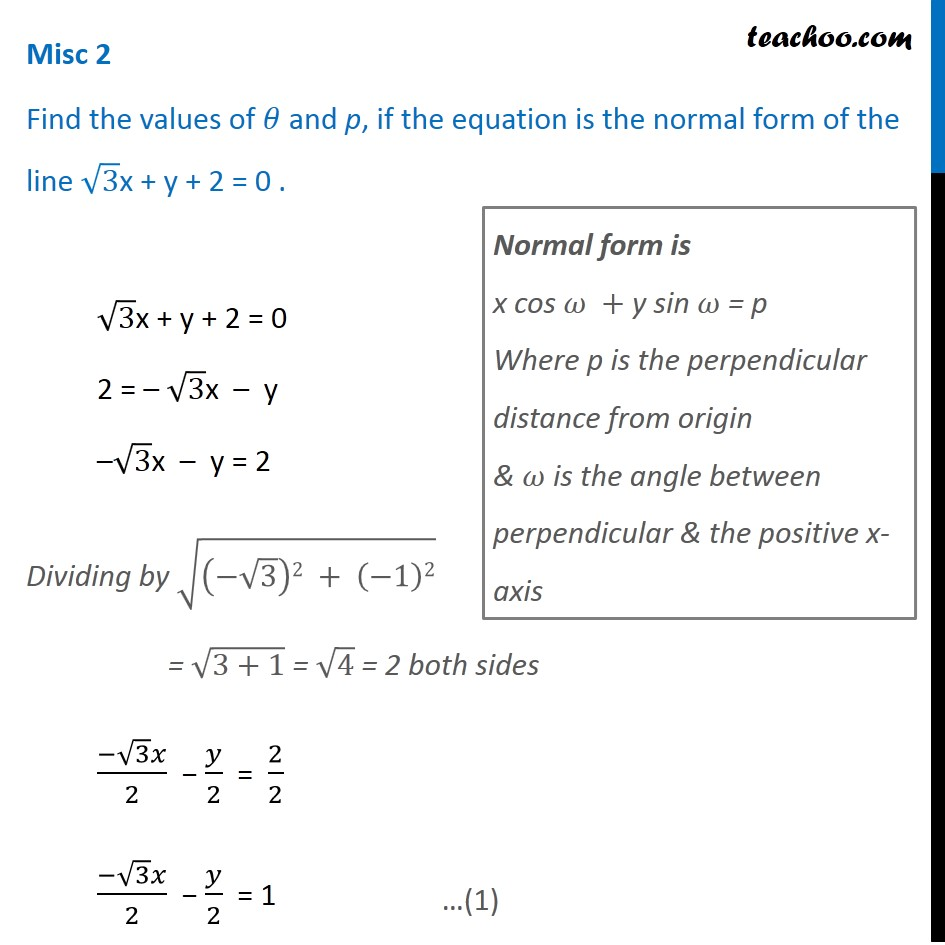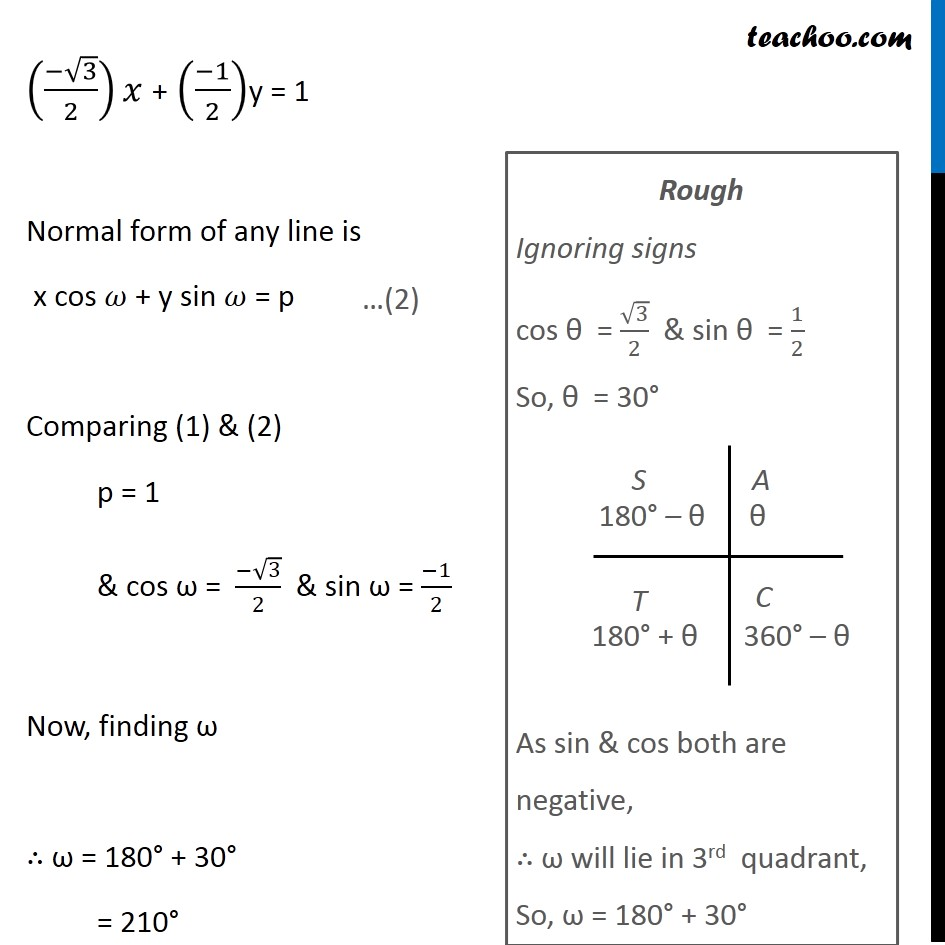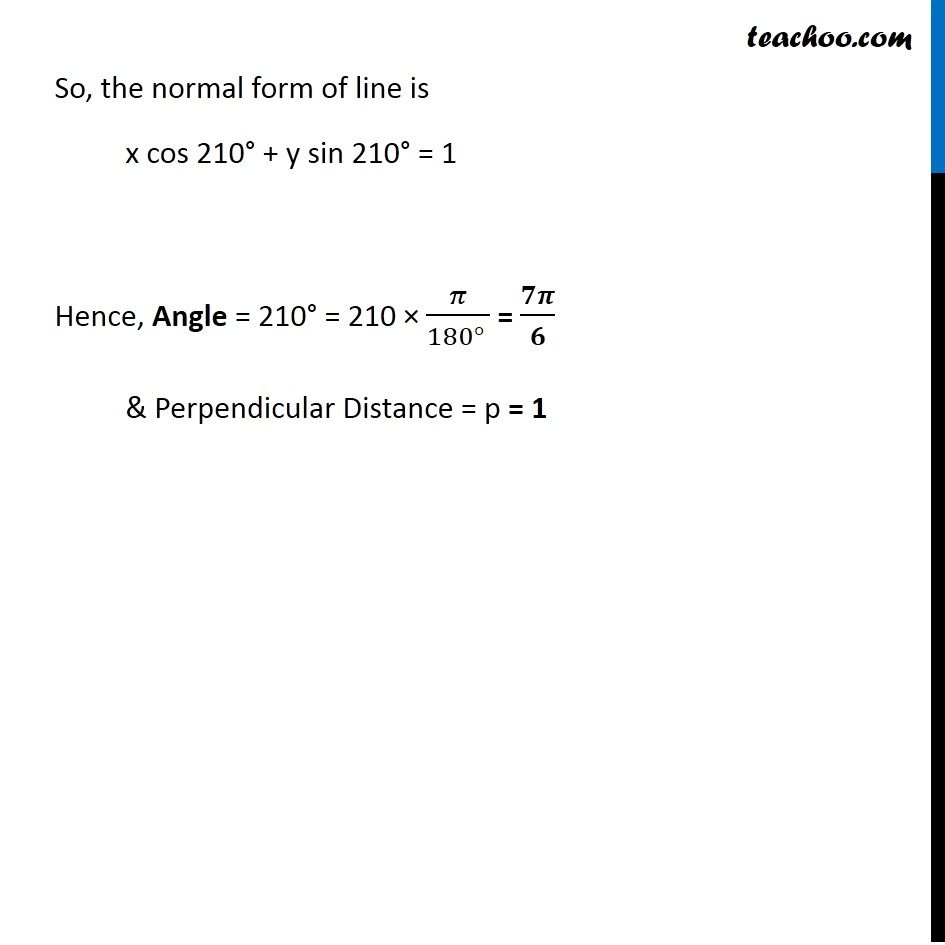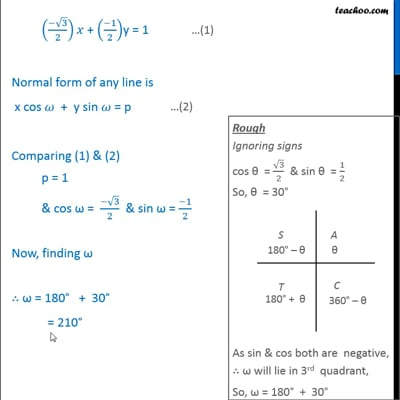Miscellaneous

Chapter 10 Class 11 Straight Lines
Serial order wiseThis video is only available for Teachoo black users

Introducing your new favourite teacher - Teachoo Black, at only ₹83 per month

### Transcript

Misc 2 Find the values of 𝜃 and p, if the equation is the normal form of the line √3x + y + 2 = 0 . √3x + y + 2 = 0 2 = – √3x – y –√3x – y = 2 Dividing by √((−√3)2 + (−1)2) = √(3+1) = √4 = 2 both sides (−√3 𝑥)/2 − 𝑦/2 = 2/2 (−√3 𝑥)/2 − 𝑦/2 = 1 Normal form is x cos 𝜔 + y sin 𝜔 = p Where p is the perpendicular distance from origin & 𝜔 is the angle between perpendicular & the positive x-axis ((−√3)/2)𝑥 + ((−1)/2)y = 1 Normal form of any line is x cos 𝜔 + y sin 𝜔 = p Comparing (1) & (2)a p = 1 & cos ω = (−√3)/2 & sin ω = (−1)/2 Now, finding ω ∴ ω = 180° + 30° = 210° Rough Ignoring signs cos θ = √3/2 & sin θ = 1/2 So, θ = 30° As sin & cos both are negative, ∴ ω will lie in 3rd quadrant, So, ω = 180° + 30° So, the normal form of line is x cos 210° + y sin 210° = 1 Hence, Angle = 210° = 210 × 𝜋/(180° ) = 𝟕𝝅/𝟔 & Perpendicular Distance = p = 1